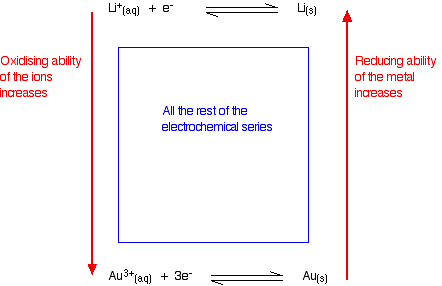Need help? Email help@shout.education

# The Electrochemical Series

This page explains the origin of the electrochemical series, and shows how it can be used to work out the ability of the various substances included in it to act as oxidising or reducing agents.

Important: If you have come straight to this page via a search engine, you should be aware that this is the second page of a linked series of pages about redox potentials. You will find it much easier to understand if you first read the introduction to redox equilibria before you go any further. A link at the bottom of that page will bring you back here again.

It is also important that you understand about redox reactions. Follow this link if you aren't confident about oxidation and reduction in terms of electron transfer.

## Building the Electrochemical Series

### Arranging Redox Equilibria in Order of Their E° Values

The electrochemical series is built up by arranging various redox equilibria in order of their standard electrode potentials (redox potentials). The most negative E° values are placed at the top of the electrochemical series, and the most positive at the bottom.

For this introductory look at the electrochemical series we are going to list the sort of metal / metal ion equilibria that we looked at on the previous page (plus the hydrogen equilibrium) in order of their E° values. This will be extended to other systems on the next page.

### The electrochemical series

equilibrium
/ V
\text{Li}^+_{(aq)} + e^- \xrightleftharpoons{} \text{Li}_{(s)} -3.03
\text{K}^+_{(aq)} + e^- \xrightleftharpoons{} \text{K}_{(s)} -2.92
\text{Ca}^{2+}_{(aq)} + 2e^- \xrightleftharpoons{} \text{Ca}_{(s)} -2.87
\text{Na}^+_{(aq)} + e^- \xrightleftharpoons{} \text{Na}_{(s)} -2.71
\text{Mg}^{2+}_{(aq)} + 2e^- \xrightleftharpoons{} \text{Mg}_{(s)} -2.37
\text{Al}^{3+}_{(aq)} + 3e^- \xrightleftharpoons{} \text{Al}_{(s)} -1.66
\text{Zn}^{2+}_{(aq)} + 2e^- \xrightleftharpoons{} \text{Zn}_{(s)} -0.76
\text{Fe}^{2+}_{(aq)} + 2e^- \xrightleftharpoons{} \text{Fe}_{(s)} -0.44
\text{Pb}^{2+}_{(aq)} + 2e^- \xrightleftharpoons{} \text{Pb}_{(s)} -0.13
2H^+_{(aq)} + 2e^- \xrightleftharpoons{} H_{2(g)} 0
\text{Cu}^{2+}_{(aq)} + 2e^- \xrightleftharpoons{} \text{Cu}_{(s)} +0.34
\text{Ag}^+_{(aq)} + e^- \xrightleftharpoons{} \text{Ag}_{(s)} +0.80
\text{Au}^{3+}_{(aq)} + 3e^- \xrightleftharpoons{} \text{Au}_{(s)} +1.50

#### A note on the hydrogen value

Remember that each E° value shows whether the position of the equilibrium lies to the left or right of the hydrogen equilibrium.

That difference in the positions of equilibrium causes the number of electrons which build up on the metal electrode and the platinum of the hydrogen electrode to be different. That produces a potential difference which is measured as a voltage.

Obviously if you connect one standard hydrogen electrode to another one, there will be no difference whatsoever between the positions of the two equilibria. The number of electrons built up on each electrode will be identical and so there will be a potential difference of zero between them.

## Oxidation / Reduction and the Electrochemical Series

### Reminders About Oxidation and Reduction

#### Oxidation and reduction in terms of electron transfer

Remember: OIL RIG

Oxidation is Loss of electrons; Reduction is Gaining of electrons

Now apply this to one of the redox equilibria:

\text{Mg}^{2+}_{(aq)} + 2e^- \xrightleftharpoons{} \text{Mg}_{(s)}

When solid magnesium forms its ions, it loses electrons. The magnesium is being oxidised.

Taking another example

\text{Cu}^{2+}_{(aq)} + 2e^- \xrightleftharpoons{} \text{Cu}_{(s)}

When the copper(II) ions gain electrons to form copper, they are being reduced.

#### Reducing agents and oxidising agents

A reducing agent reduces something else. That must mean that it gives electrons to it.

Magnesium is good at giving away electrons to form its ions. Magnesium must be a good reducing agent.

An oxidising agent oxidises something else. That must mean that it takes electrons from it.

Copper doesn't form its ions very readily, and its ions easily pick up electrons from somewhere to revert to metallic copper. Copper(II) ions must be good oxidising agents.

### Summarizing This on the Electrochemical Series

Metals at the top of the series are good at giving away electrons. They are good reducing agents. The reducing ability of the metal increases as you go up the series.

Metal ions at the bottom of the series are good at picking up electrons. They are good oxidising agents. The oxidising ability of the metal ions increases as you go down the series.### Judging the Oxidising or Reducing Ability from E° Values

The more negative the E° value, the more the position of equilibrium lies to the left – the more readily the metal loses electrons. The more negative the value, the stronger reducing agent the metal is.

The more positive the E° value, the more the position of equilibrium lies to the right – the less readily the metal loses electrons, and the more readily its ions pick them up again. The more positive the value, the stronger oxidising agent the metal ion is.